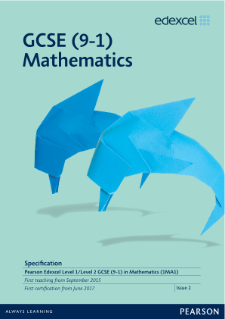# GCSE MATHEMATICS RETAKE

Students will expand on their existing knowledge and learn about the various areas of mathematics, such as number, algebra, geometry, ratio and proportion, probability and statistics. Students will study towards a GCSE Mathematics qualification provided by Edexcel at either Higher or Foundation level.

 YEAR 12 TERM 1 Numerical skills  HCF/LCM  Fractions  Decimals  Percentages  Ratio & Proportion  Standard form & Indices  Expressions  Equations  Graphs  Sequences  Linear inequalities  Formulae TERM 2 Angles  Transformations  Area & Volume  Pythagoras theorem  Trigonometry  Similar shapes  Properties of circle  Vectors  Probability  Cumulative frequency  Box plots TERM 3 Revision of key skills and examination practice

For more detailed information click on the icon below: